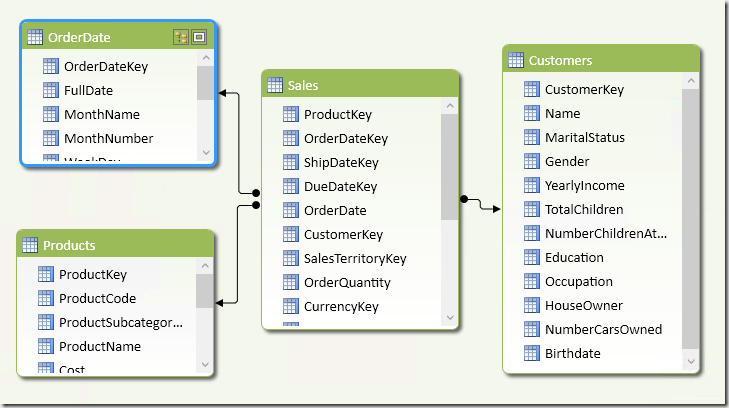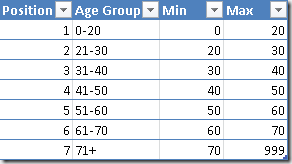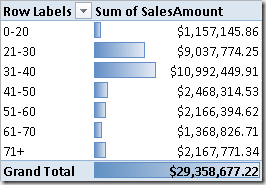This scenario would be easier to implement if you want to consider the current age of Customer for any transaction she made in the past. However, if you have a transactions history that spans over several years, a better analysis is performed by considering the age of the customer at the moment of every transaction. With a large volume of data, in a star schema this requires the creation of a separate dimension just for the age of the customer, adding a column in the fact table that persists that age. This result in an expensive cost also for Analysis Services Multidimensional, because it increases cube’s dimensionality.

In PowerPivot and Analysis Services Tabular models, you can create a calculated column that persists the age of the customer for each transaction with a simple DAX formula. In the following schema, every customer has a Birthdate column and every transaction in Sales has an OrderDate column, both containing dates.In the Sales table you can create a CustomerAge column with the following DAX expression:

```CustomerAge =
YEAR ( Sales[OrderDate] )
- YEAR ( RELATED ( Customers[Birthdate] ) )
+ IF (
DATE (
1900,
MONTH ( Sales[OrderDate] ),
DAY ( Sales[OrderDate] )
) < DATE (
1900,
MONTH ( RELATED ( Customers[Birthdate] ) ),
DAY ( RELATED ( Customers[Birthdate] ) )
),
- 1,
0
)```

The following step is creating an AgeGroups table that defines the age groups you want to use in a PivotTable. For example, the following table can be used as linked table in PowerPivot, or it could be a regular table in a Tabular model.You cannot create a direct relationship between Sales and AgeGroups, because you do not have a row in AgeGroups for every value of CustomerAge in Sales table. Moreover, such a relationship would be expensive considering we only need to group values in a smaller number of combinations (7 in this example). Thus, a better optimization is denormalizing the Age Group column in the fact table, doing the same for the Position column in order to apply the Sort by Column property to Age Group.

The Age Group column in Sales table can be defined with the following formula, which gets the only value of Age Group of the corresponding age interval. In case of a bad configuration in AgeGroups table, with overriding intervals, you would get an error during table refresh.

```AgeGroup =
CALCULATE (
VALUES ( AgeGroups[Age Group] ),
FILTER (
AgeGroups,
Sales[CustomerAge] >= AgeGroups[Min]
&& Sales[CustomerAge] < AgeGroups[Max]
)
)```

The AgeGroupPosition column has a similar definition, changing only the column returned from AgeGroups. This column will be hidden and used only for the sort order of AgeGroup.

```AgeGroupPosition =
CALCULATE (
VALUES ( AgeGroups[Position] ),
FILTER (
AgeGroups,
Sales[CustomerAge] >= AgeGroups[Min]
&& Sales[CustomerAge] < AgeGroups[Max]
)
)```

You can use the AgeGroup column in a PivotTable like in the following example, which splits SalesAmount by groups of customers’ age.This pattern works very well, but it can be further optimized. We added 3 columns (CustomerAge, AgeGroup and AgeGroupPosition) in the Sales table. The CustomerAge column contains usually around 100 distinct values, which can be considered a good compression, but the other two columns only contains 7 distinct values and requires just 3 bits instead of 7. Thus, removing the CustomerAge column might produce a saving of 50% of space required for adding this analysis feature. You have to rewrite the AgeGroup and AgeGroupPosition columns in this way in order to eliminate the dependency from CustomerAge, which you can remove then.

```AgeGroup2 =
CALCULATE (
VALUES ( AgeGroups[Age Group] ),
FILTER (
AgeGroups,
(RELATED( Customers[Birthdate] )
>= DATE ( YEAR ( Sales[OrderDate] ) - AgeGroups[Max], MONTH ( Sales[OrderDate] ), DAY( Sales[OrderDate] ) )
|| AgeGroups[Max] > 500)
&& RELATED( Customers[Birthdate] )
< DATE ( YEAR ( Sales[OrderDate] ) - AgeGroups[Min], MONTH ( Sales[OrderDate] ), DAY( Sales[OrderDate] ) )
)
)

AgeGroupPosition2 =
CALCULATE (
VALUES ( AgeGroups[Position] ),
FILTER (
AgeGroups,
(RELATED( Customers[Birthdate] )
>= DATE ( YEAR ( Sales[OrderDate] ) - AgeGroups[Max], MONTH ( Sales[OrderDate] ), DAY( Sales[OrderDate] ) )
|| AgeGroups[Max] > 500)
&& RELATED( Customers[Birthdate] )
< DATE ( YEAR ( Sales[OrderDate] ) - AgeGroups[Min], MONTH ( Sales[OrderDate] ), DAY( Sales[OrderDate] ) )
)
)```

Even it the DAX code of the last example is longer and slightly slower, it is executed only at table refresh time, saving precious memory resource if the Sales table contains millions of rows.